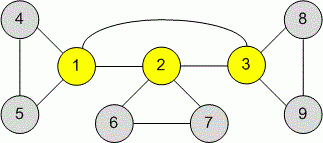Competitions

# Articulation Points

The undirected graph is given. Find all its articulation points.

#### Input

The first line contains two positive integers n and m (n2 * 104, m2 * 105) - the number of vertices and edges respectively.

Each of the next m lines contains the description of an edge. The edge number i is given with two positive integers bi, ei (1bi, ein) - the numbers of the vertices it connects.

#### Выходные данные

Print in the first line the number b of articulation points in a given graph. In the next b lines print the numbers of the vertices that are articulation points in increasing order.Time limit 3 seconds
Memory limit 128 MiB
Input example #1
9 12
1 2
2 3
4 5
2 6
2 7
8 9
1 3
1 4
1 5
6 7
3 8
3 9
Output example #1
3
1
2
3

Author Vitaly Goldstein
Source Winter School, Kharkov, 2011, Day 9